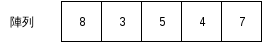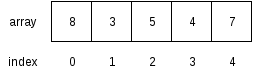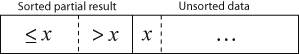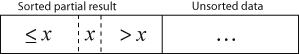# 陣列 (Array)、向量 (Vector) 和切片 (Slice)

## 前言

先前的程式中，變數僅表示單一的實體 (entity) 我們從本章開始，會介紹數種容器 (collections)，容器有特定的內部結構，其作用在於裝載資料，此外，容器會提供一些方法，讓我們藉由操作容器，存取其中的資料。傳統上，容器相關的內容多見於介紹資料結構 (data structure) 的書籍，有興趣的讀者可自行查閱相關資料。本章會介紹陣列 (array)、向量 (vector) 和切片 (slice)。

## 陣列

陣列是一種線性的容器，儲存同一種型別的資料，而其長度在生成後即固定下來，陣列中的資料在記憶體中是連續而緊密排列的，如下圖：陣列的好處在於可快速存取陣列中的資料，因為陣列是透過索引值存取資料，但當要改變陣列長度時，效率則較差，因為要複製陣列中的資料。

### 建立陣列

建立陣列有兩種方式，一種是直接將資料寫在陣列中，如以下範例：

``````fn main() {
let array = [1, 2, 3, 4, 5];
}
``````

一種則是以 `[T; N]` (T: 型別，N: 長度) 這種方式初始化陣列。範例如下：

``````fn main() {
const SIZE: usize = 5;

// [0.0, 0.0, 0.0, 0.0, 0.0]
let mut array: [f64; SIZE] = [0.0; SIZE];
}
``````

C 或 C++ 可用動態配置記憶體産生陣列，在 Rust 中相對應的動作是使用向量 (vector)。向量是一種可動態改變大小的線性容器，我們將於下文介紹。

### 存取陣列資料

陣列使用非負整數作為存取資料的索引 (index)，如下例：

``````fn main() {
let array = [1, 2, 3];

assert_eq!(array, 1);
assert_eq!(array, 2);
assert_eq!(array, 3);
}
``````

要注意的是，陣列的索引值是從 0 開始，對於程式設計初學者來說，時常會覺得容易搞混。一個簡單的想法是將索引值視為偏離值 (offset)，如下圖：也可將資料存入陣列，如下例：

``````fn main() {
let mut array = [0; 3];

array = 1;
array = 2;
array = 3;

assert_eq!(array, 1);
assert_eq!(array, 2);
assert_eq!(array, 3);
}
``````

### 走訪陣列

使用陣列等容器的好處之一，在於可以結合迴圈走訪陣列中的資料。如果我們想走訪陣列中的元素，其中一個方法是以陣列的索引值來走訪陣列，如下例：

``````fn main() {
// Numbers in German
let array = ["eins", "zwei", "drei", "vier", "fünf"];

for i in 0..array.len() {
println!("{}", array[i]);
}
}
``````

或是使用迭代器，如下：

``````fn main() {
let array = ["eins", "zwei", "drei", "vier", "fünf"];

for element in array.iter() {
println!("{}", element);
}
}
``````

然而，陣列本身不能走訪，所以以下程式碼是錯誤的：

``````fn main() {
let array = ["eins", "zwei", "drei", "vier", "fünf"];

// Error
for element in array {
println!("{}", element);
}
}
``````

會引發以下錯誤訊息：

``````error[E0277]: the trait bound `[&str; 5]: std::iter::Iterator` is not satisfied
``````

如果要在走訪陣列時，修改其中的資料，可用索引值走訪陣列，如下：

``````// Call rand package for random number generation
extern crate rand;

use rand::Rng;

fn main() {
const SIZE: usize = 10;
let mut array = [0; SIZE];

for i in 0..array.len() {
// Set a random number between 1 and 100
array[i] = rand::thread_rng().gen_range(1, 100 + 1);
}
}
``````

如果要使用迭代器，則可修改程式如下：

``````extern crate rand;

use rand::Rng;

fn main() {
const SIZE: usize = 100;
let mut array = [0; SIZE];

for e in array.iter_mut() {
*e = rand::thread_rng().gen_range(1, 100 + 1);
}
}
``````

其中的 `*e` 用到參考 (reference) 的概念，簡單地說，參考存的是變數在記憶體中位置，我們透過解參考 (dereferencing) 取得變數本身。我們會於後續章節中介紹參考。

### 陣列的限制

目前 Rust 的陣列有一些使用上的限制，某些函式在陣列長度大於 32 時無法使用。像是下列看起來正常無誤的程式碼：

``````extern crate rand;

use rand::Rng;

fn main() {
const SIZE: usize = 33;  // Watch out when SIZE > 32
const MIN: i32 = 1;
const MAX: i32 = 100;

let mut array: [i32; SIZE] = [0; SIZE];

for i in 0..SIZE {
array[i] = rand::thread_rng().gen_range(MIN, MAX + 1);
}

println!("{:?}", array);  // Error when SIZE > 32
}
``````

卻引發下列錯誤：

``````error[E0277]: the trait bound `[i32; 33]: std::fmt::Debug` is not satisfied
``````

這些 trait 的大小限制是 Rust 內部實作的問題，而不是一般程式語言中陣列的正常行為，Rust 官方文件也有提到這個議題。在 Rust 改善這點前，我們有幾個處理方式，包括：

1. 自行實作相關 trait
2. 避免使用這些方法
3. 改用向量

(1) 不是通用的方法，因為針對每個長度，都要重新實作一次，但若有需求，仍可考慮；(2) 則會限制了陣列的使用場合；通常可考慮 (3)。

## 向量

向量 (vector) 是一種可動態改變長度的線性容器，其內部實作也是陣列，但可動態增加長度。由於向量使用方式類似陣列，但較陣列靈活，實際上，向量使用的場合會比陣列多。

### 建立向量

建立向量有兩種方式，一種是以 `vec!` 巨集直接建立，一種是先建立空的向量後再陸續加入資料。

以下程式以 `vec!` 巨集建立 vector：

``````fn main() {
let vec = vec![1, 2, 3];
}
``````

以下程式先建立向量後，再加入資料：

``````fn main {
/* Type inference works here,
so we don't explicitly declare
the type of vec. */
let mut vec = Vec::new();  // vec<i32>

// Append data into the tail of the vector
vec.push(1);
vec.push(2);
vec.push(3);
}
``````

`push` 的概念是，從向量尾端附加一個新的元素，就像是在一列火車尾端掛載一節車箱般。Rust 的向量從尾端加入資料的效率相當好，可視為常數時間。

註：以演算法的術語來說，為 amortized O(1)。

### 存取向量資料

和陣列類似，向量也是以非負整數做為索引。見以下範例：

``````fn main() {
let vec = vec![1, 2, 3];

assert_eq!(vec, 1);
assert_eq!(vec, 2);
assert_eq!(vec, 3);
}
``````

同樣地，向量也可以存入資料。如下例：

``````fn main() {
let mut vec = vec![1, 2, 3];

vec = 99;  // Feed data into vector

assert_eq!(vec, 1);
assert_eq!(vec, 99);
assert_eq!(vec, 3);
}
``````

### 走訪向量

類似於陣列，如果要走訪向量，可以使用數字為索引，如下例：

``````fn main() {
let vec = vec![1, 2, 3];

for i in 0..(vec.len()) {
println!("{}", i);
}
}
``````

或是使用迭代器，如下例：

``````fn main() {
let vec = vec![1, 2, 3];

for element in vec.iter() {
println!("{}", element);
}
}
``````

如果需要在走訪向量時改變其值，可以用索引走訪：

``````fn main() {
let mut vec = vec![1, 2, 3];

for i in 0..vec.len() {
vec[i] = vec[i] * vec[i];
}

assert_eq!(vec, 1);
assert_eq!(vec, 4);
assert_eq!(vec, 9);
}
``````

或是使用迭代器：

``````fn main() {
let mut vec = vec![1, 2, 3];

for element in vec.iter_mut() {
*element = (*element) * (*element);
}

assert_eq!(vec, 1);
assert_eq!(vec, 4);
assert_eq!(vec, 9);
}
``````

同樣地，這裡用到解參考。

### 一些操作向量的方法

以下用實例來介紹操作向量的方法 (methods)：

``````fn main() {
// Declare an empty vector
let mut vec = Vec::new();

// Append data to the tail of the vector
vec.push(1);
vec.push(2);
vec.push(3);

// Get the length of the vector
assert_eq!(vec.len(), 3);

// Pop data from the tail of the vector
let popped = vec.pop().unwrap();
assert_eq!(popped, 3);
assert_eq!(vec, vec![1, 2]);

// Insert data into the middle of the vector
vec.insert(1, 99);
assert_eq!(vec, vec![1, 99, 2]);

// Remove data from the middle of the vector
let removed = vec.remove(1);
assert_eq!(removed, 99);
}
``````

由本例，可以看到向量可動態改變長度，而不需手動進行資料的搬移。然而，向量內部仍然是數列，除了從尾端增加資料外，向量在增減長度時會牽涉到資料的拷貝，若有大量資料搬移的需求，可能要考慮改用其他的容器。

`vec.pop().unwrap()` 這個部分的程式碼可能會令讀者困惑，這是 Rust 的特殊容器 Option。該容器的用途是為了處理錯誤情形，在從向量尾端取出資料時，有可能取出的值為空值，故 Rust 將值包裝在該容器中。這部分牽涉到 enum 的概念，將於後續章節中說明。

## 切片

切片 (slice) 是一種用來間接存取陣列或向量的元素的型別，其內部包括指向陣列或向量的參考和原本的陣列或向量的長度。由於向量使用參考，故不需要拷貝陣列或向量的資料。簡單地說，切片不存放資料本身，而存放指向資料的記憶體位置，透過切片，可間接取得資料。

### 建立切片

建立切片的方法是先建立陣列或向量後，再建立切片，如下例：

``````fn main() {
/* Internally, it works as this:
let _slice = [1, 2, 3, 4, 5];
let slice = &_slice; */
let slice = &[1, 2, 3, 4, 5];
}
``````

有 C 或 C++ 經驗的讀者可能會覺得困惑，為什麼我們可以對值取參考。其實，在內部，Rust 會建立一個暫時變數，再將其參考指向程式設計者指定的變數。

### 存取切片資料

如同陣列，切片也可以用索引取出資料，如下例：

``````fn main() {
let slice = &[1, 2, 3];

assert_eq!(slice, 1);
assert_eq!(slice, 2);
assert_eq!(slice, 3);
}
``````

若設定適當的權限，切片也可寫入資料，如下例：

``````fn main() {
let slice = &mut [1, 2, 3];

// Write data into slice
slice = 99;

assert_eq!(slice, 1);
assert_eq!(slice, 99);
assert_eq!(slice, 3);
}
``````

### 走訪切片

切片的其中一個作用，在於可自動轉為迭代器，範例如下：

``````fn main() {
let array = ["eins", "zwei", "drei", "vier", "fünf"];

// It works when the array size <= 32
for element in &array {
println!("{}", element);
}
}
``````

如果切片是由陣列而來，而原陣列元素個數在超過 32 個時，此方法不能使用，見前文說明。經筆者測試，若切片是由向量轉來，則沒有上述限制。

設定好權限後，也可以在走訪切片時改變其值，範例如下：

``````extern crate rand;

use rand::Rng;

fn main() {
const SIZE: usize = 10;
let mut array = [0; SIZE];

for element in &mut array {
*element = rand::thread_rng().gen_range(1, 100 + 1);
}
}
``````

如前述，若切片是由陣列而來，同樣會受到長度限制的問題。

## (案例選讀) 插入排序法

在本節中我們練習實作插入排序法 (insertion sort)。插入排序法是一種簡單易懂的排序演算法 (sorting algorithm)，對於小型的資料效率佳。實作方式有兩種，一種是額外建立一個串列，再將資料依序插入該串列中，一種則是原地修改陣列，本節採用後者。

陣列在排序前如下示意圖 (摘自維基百科)：而排序後如下圖 (摘自維基百科)：將插入排序法寫成虛擬碼如下：

``````Let A a zero-based array with size n

for i from 1 to n - 1 {
x = A[i]
j = i
while j > 0 and A[j-1] > x {
A[j] = A[j-1]
j = j - 1
}
A[j] = x
}
``````

同樣地，我們的範例程式碼用到 rand 套件，需於 Cargo.toml 中加入相關內容。

這裡附上範例程式碼，以供參考：

``````// Call rand library
extern crate rand;

use rand::Rng;

fn main() {
// Initialize variables
const SIZE: usize = 10;
let mut array: [i32; SIZE] = [0; SIZE];

// Set array elements with random integers
for i in 0..SIZE {
array[i] = rand::thread_rng().gen_range(1, 100 + 1);
}

// Print out unsorted array
print!("Before sort: ");
display_slice(&array);

// Insertion sort.
// Modify the array in-place.
for i in 1..(array.len()) {
let x = array[i];  // Temp data
let mut j = i;
while j > 0 &&  array[j-1] > x {
array[j] = array[j-1];  // Move element one step
j -= 1;
}
array[j] = x;  // Put back temp data
}

// Print out sorted array
print!("After sort: ");
display_slice(&array);
}

// Function to print out array with arbitrary size
fn display_slice(slice: &[i32]) {
for i in 0..slice.len() {
print!("{}", slice[i]);

if i < slice.len() - 1 {
print!(", ");
}
}
println!("");
}
``````

在這裡，我們暫不講解關於函式的部分，而留在後續章節中另行介紹。簡單地說，這個函式不受到陣列長度的限制，可在終端機印出切片內的資料。對於有 C 程式設計經驗的讀者，會發現該函式接收切片後可從切片得到其長度，在內部，Rust 的切片和 C 的陣列不同，帶有長度的資訊。

除了插入排序法外，還有一些排序演算法可以用陣列實作，舉例如下：

• Bubble sort
• Selection sort
• Bucket sort

讀者有興趣的話，可自行試著練習看看這些演算法。

【分享本文】